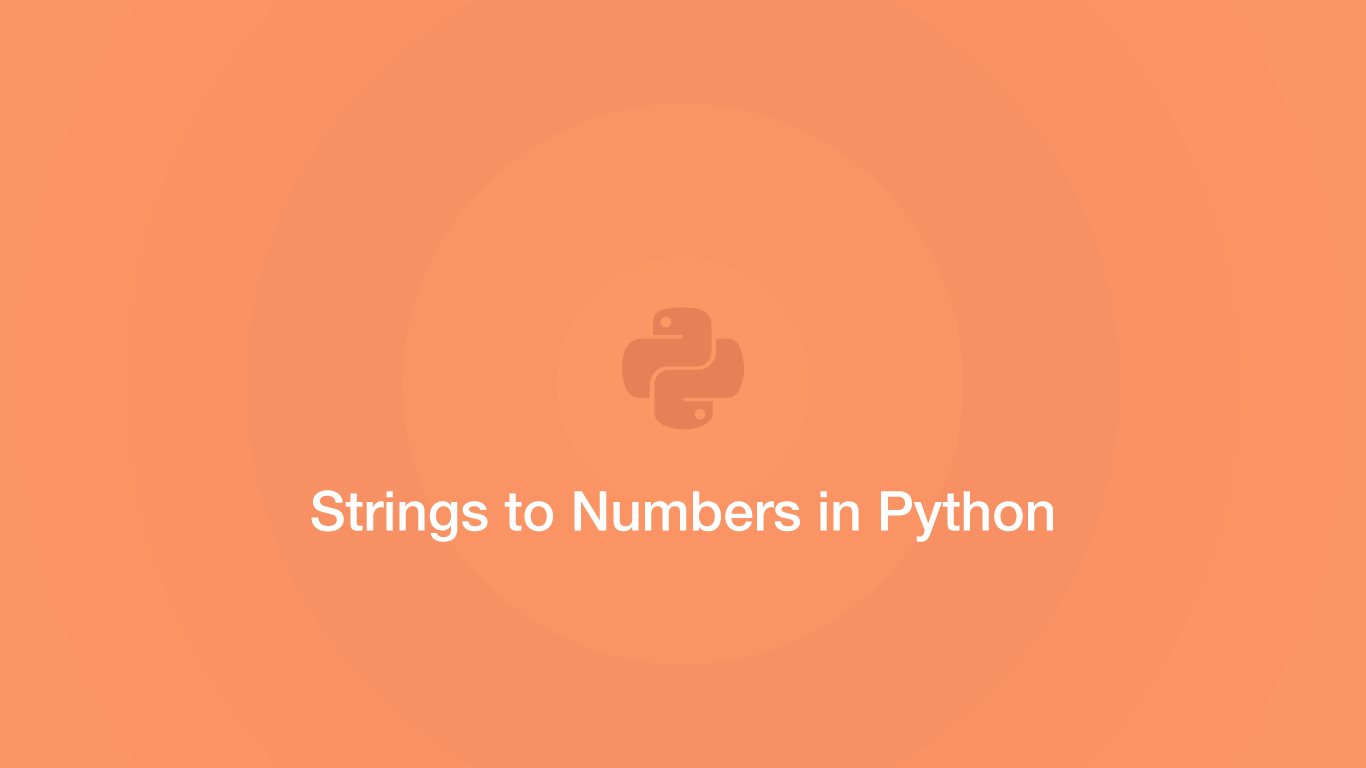# How to Convert a String to a Number in PythonSometimes we will need to convert a string with a numerical value into a number in Python. Fortunately, Python provides built-in functions for converting strings into integers and floats.

In this tutorial, we will learn how to convert strings to numbers in Python.

## The int() Function

To convert a numerical string to an integer use the `int()` function, passing the string to convert inside the `()` (parenthesis) of the function.

``````string = '15'

num = int(string)

print(type(num))
``````
``````<class 'int'>
``````

In the above example, we are evaluating the type of data the string has been converted to using the `type()` function.

note - if the input string has a floating-point numerical value or any other non-numerical values, `int()` won't be able to convert it and a `ValueError` will be thrown:

``````string = '15.1'

num = int(string)

print(type(num))
``````
``````---------------------------------------------------------------------------
ValueError                                Traceback (most recent call last)
<ipython-input-20-70832f88df42> in <module>
1 string = '15foo'
2
----> 3 num = int(string)
4
5 print(type(num))

ValueError: invalid literal for int() with base 10: '15.1'
``````

## The float() Function

To convert a string that contains a floating-point number we can use the `float()` function, passing the string inside the `()` (parenthesis).

note - if the number is not a float or an integer, `float()` will throw a `ValueError` exception:

``````string = '15bar'

num = float(string)

print(type(num))
``````
``````---------------------------------------------------------------------------
ValueError                                Traceback (most recent call last)
<ipython-input-25-75435004e715> in <module>
1 string = '15bar'
2
----> 3 num = float(string)
4
5 print(type(num))

ValueError: could not convert string to float: '15bar'
``````

## Checking a String is a Number in Python

The above two examples only work if we assume the input string will always contain only an integer or a float. If this is not the case a `ValueError` will be thrown. To check if all the characters in a string are numerical and therefore an integer use the `isnumeric()` method.

``````string = '15bar'

if string.isnumeric():
num = int(string)

print(num)
else:
print('Number contains non numerical characters')
``````
``````Number contains non numerical characters
``````

## Checking a String is a Float in Python

The only non-numerical character that a floating-point number contains is a `.` (dot). Therefore we can replace all instances of `.` (dot) with nothing using the `replace()` method before checking it with the `isnumeric()` method.

``````string = '15.1'
no_dot = string.replace('.', '')

if no_dot.isnumeric():
num = float(string)

print(num)
else:
print('Number is not a float')
``````
``````15.1
``````

## Converting a Number to a String

If the number needs to be converted back into a string, use the `str()` function, supplying the integer or float inside the `()` parenthesis of the function.

``````num = 15

string = str(num)

print(type(string))
``````
``````<class 'str'>
``````

## Printing a Number in a String

A number can't be concatenated inside a string like this:

``````num = 0

print('The number is: ' + num + '')
``````
``````---------------------------------------------------------------------------
TypeError                                 Traceback (most recent call last)
<ipython-input-11-a2b6c9d13ae3> in <module>
1 num = 0
2
----> 3 print('The number is: ' + num + '')

TypeError: can only concatenate str (not "int") to str
``````

The cleanest way to get around this to use an f string, which can accept numbers inside `{}` (curly brackets).

``````num = 0

print(f'The number is: {num}')
``````
``````The number is: 0
``````

## Conclusion

You now know how to convert strings containing numerical values into floating-point numbers and integers in Python.

#### Related Tutorials### How to Convert a List to a String in Python

September 17, 2020### How to Compare Strings in Python

September 20, 2020### How to Get Inputs in Python

October 01, 2020### How to use Python datetime to Create and use Dates and Times

September 24, 2020### How to Parse JSON Data in Python (Read and Write)

September 18, 2020### How to Use Argparse in Python

September 25, 2020